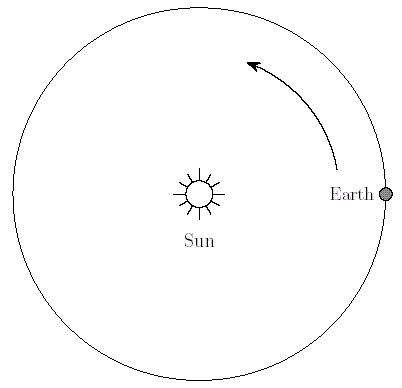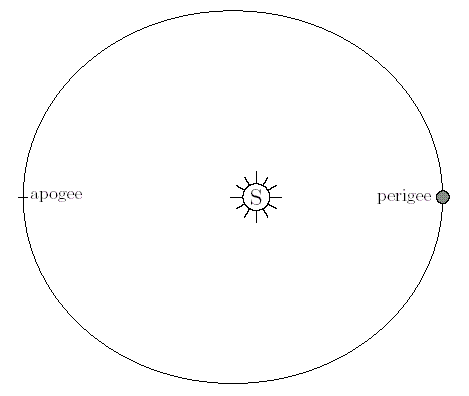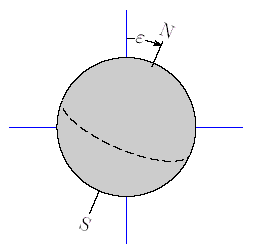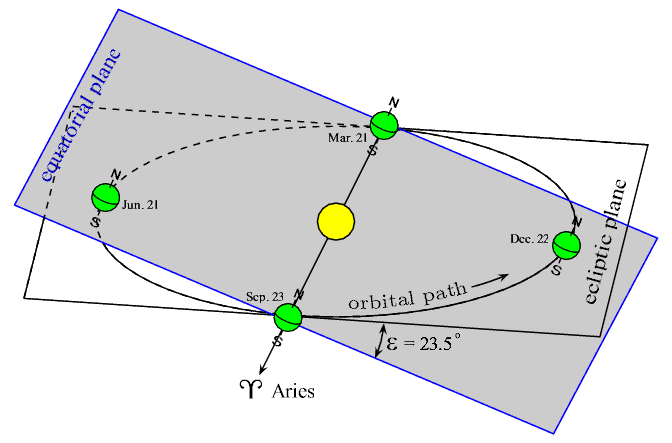﻿ Channel

# Astronomy Algorithms and Software

Astronomy is an ideal science for computer applications. Not only does the computer provide an essential tool for astronomical and orbital calculations but also in the analysis and display of celestial images.## A Gentle Introduction to the Positional Astronomy of the Solar System

### The Solar System

The ancients believed that all Solar and celestial phenomena could be described in terms of perfect spheres and circles. With the invention of the telescope and increasingly accurate measurement tools, we have found this perfection to be illusory. In fact, an accurate description of the position of any of the planets may require hundreds of adjustments to account for perturbations due to the presence of moons or other planets, gyroscopic effects, aberration, parallax, refraction, etc.

Fortunately, it is possible to solve the problem of determining the position of the Sun or planets at any time by starting with a very simple model and making adjustments for perturbations as needed until sufficient accuracy is achieved. This process of stepwise refinement can be terminated early for reduced accuracy or continued as needed.

### Solar Model

Our first project is to find a way to determine the position of the Earth with respect to the Sun at any time. We will sometimes pretend that the Sun moves around the Earth, as the ancients thought, and refer to the position of the Sun with respect to the Earth. For our limited purposes, the difference in perspective is not material.

We begin our study with the simplest possible model of the Solar system consisting of only the Sun and Earth. All other planets are ignored in this model, and we start with the assumption that the Earth orbits the Sun in a perfectly circular orbit. As already noted, this simplification does not hold up under close examination, but it serves the purpose of establishing a starting point.A Simple Model of the Earth/Sun System

For this model, the Sun is at the center of the Solar system and the Earth orbits it in a heliocentric (sun centered) orbit with uniform angular motion (constant speed). A simple equation describing the motion is

q t = q0 + w t

qt  is the current angular displacement from some reference position, q0.  w is the angular velocity in degrees or radians per second and t is the elapsed time from any previous passage through q0.

Clearly, for this model to be useful, we must have some way of determining a reference position at a reference time. The circular orbit shown is a continuous, unending curve and does not exhibit any properties which could be used to identify a reference position. Fortunately, the real solar system exists within a more or less fixed celestial context. Thus it is possible to draw an imaginary line from the center of the Earth through the Sun and on to some celestial object at some point in time and use this line as an orbital position reference. Whenever the Earth, Sun and reference object line up, the Earth has completed a revolution around the Sun.

### Fundamental Reference Constants

Fortunately, reference positions and times for the relation of the Earth to the Sun have been determined with high accuracy. Unfortunately, the values obtained are referred to phenomena which do not exhibit exactly 'constant' behavior. Hence, these positions and times are subject to recalculation periodically as the motion of the solar system evolves. Nevertheless, the accepted framework for calculating the relation of the Earth to the Sun at any time is sufficiently stable that it is adequate for short term use.

NOTE: The empirical constants which appear in the following paragraphs are expressed in much higher precision than is normally required. For applications requiring only low to moderate precision the constants can be rounded to 3 or 4 significant digits.

### Length of year

In the late 1950's an international astronomical committee met to establish standard values for some fundamental measured quantities. The value accepted for the length of the specific tropical year beginning 1900 January 0.5 adopted by the committee is:

31 556 925.9747 (ephemeris) seconds

The tropical year will be explained in more detail later, but for the moment we note that this is the elapsed time between successive passages of the Sun through the reference position known as the vernal equinox. The number of days in a tropical year can be found by dividing this number by the number of seconds in a day.

31 556 925.9747 ÷ 86400 = 365.242198781 days

The fractional part of this number reminds us that the earth does not make an exact number of rotations in a year. Interestingly, if there were exactly 365 days in a year, the earth would have actually made exactly 366 revolutions.

We can now use this information to flesh out the equation describing the Earth's motion. If we are interested in the motion described by the simple model above, and want to determine the number of degrees the Earth has moved from the reference position q0 , in t days, we take the angular velocity as: w = 360 ÷ 365.242198781 = 0.985647335 degrees per day. Recall that the accepted reference position is associated with the vernal equinox and for this model represents the angle the Earth covers from its previous passage through that point until its position at Jan. 0.5, 1900. This strange date and date format will be explained in later paragraphs. For the moment, note that it represents midnight  between the previous December 31 and January 1.

### Reference position

The angular position of the mean vernal equinox preceding Jan. 0.5, 1900 has also been determined with high accuracy. For t = 0, at Jan 0.5, 1900 the Earth had traversed 279.69667778 degrees from this reference. Hence, the formula for the Earth's angular position at any time can be given by,

qt = 279.69667778 + 0.985647335 t

where t is the number of (Julian) days elapsed since Jan 0.5, 1900. We will use decimal degrees exclusively to measure angles, but note that most publication on astronomy use both decimal degrees and degrees-minutes-seconds format.

The position calculated above is an angular displacement from the mean vernal equinox of the reference date. The calculated position is an approximation to the mean solar longitude. Bear in mind that our model is simplified to the point that it is not sufficiently accurate for many applications. The precision of the constants suggests a much higher accuracy than that actually attainable by the model. We will improve the model later, but for now note that we do have an approximate equation describing the progress of the Earth's orbit around the Sun.

It is worth reminding the reader that we are interested in the position of the Earth in its progress around the Sun, but that it is often referred to as if the Sun were orbiting the Earth. In fact, it really doesn't make any difference which point of view is taken. The equations are ultimately the same.

### Eccentricity of Orbit

The first correction to the simplified model is to replace the perfectly circular orbit with a slightly elliptical one. Newton provided us with reasons to expect orbiting bodies to follow elliptical paths and the measurements taken before and after his time confirm it. The eccentricity, which is a measure of the departure from a circle, is small for the Earth's orbit.

The illustration below shows the Earth following a mildly elliptical orbit with the nearest, perigee, and farthest, apogee,  points from the Sun indicated.Elliptical orbit with perigee and apogee.

The ratio of the distance at apogee to that at perigee can be found from the expression (1+e) / (1-e), where e is the eccentricity. This expression is valid whenever e varies between 0 and 1. The eccentricity of the Earth's orbit at the Jan. 0.5, 1900 was:

e = 0.01675105

The ratio is then 1.034073, revealing that there is only about a 3.4% difference between the greatest and least distances. This is small, but will  be used later to in improve the model for better accuracy.

Incidentally, the terms, perigee and apogee, are generally used to refer to distance between the Earth and an orbiting satellite. In the case of a planet's distance from the Sun, it is customary to use the terms, perihelion and aphelion

### Plane of the Ecliptic

The plane in which the orbit of the Earth around the Sun lies is called the ecliptic plane or simply the ecliptic.

### Equatorial Plane

If the Sun and Earth were the only objects in the universe, and if the Earth followed an exactly circular orbit around the Sun, and if the axis around which the Earth rotates daily was exactly perpendicular to the plane of the Earth's orbit around the Sun, it would be difficult to establish a reference position for positional astronomy. On the other hand, if those conditions existed there would probably be no interest or value in computing the Earth's position.

In the physical solar system, the Earth's orbit is not exactly circular, but elliptical, as has been discussed. Furthermore, the axis of the Earth's daily rotation is not perpendicular to the ecliptic. Hence, the plane defined by the Earth's equator is tilted from the ecliptic by an angle referred to as the obliquity of the ecliptic. The symbol used to signify this angle is the Greek letter epsilon, e. At Jan. 0.5, 1900 its value was:

e = 23.452294oTilt of the Earth's Axis (e = 23.5o)

The two planes defined by the system described so far are sometimes called the orbital plane and the equatorial plane. From plane geometry we know that two planes are either parallel or else they intersect in a straight line. In this case, there must be an intersection and the resulting line will pass through the Sun whenever the line from the center of Sun through the center of the Earth cuts the equator. A diagram illustrating this is presented in the next section.

### Vernal Equinox

In the figure below, we see the relation between the equatorial plane and the orbital plane (ecliptic). The Earth is shown at four positions during the year for reference.Diagram of Earth's Motion Around the Sun

There are several ways to interpret the orientation of the planes and their line of intersection. If we assume that the equatorial plane stays fixed through the Earth's equator, then two times a year the line of intersection with the orbital plane passes through the Sun. If we assume that the planes are fixed so that their line of intersection always passes through the Sun , then two times a year the Earth crosses this line. It doesn't matter which point of view is taken, because the two events under consideration occur at exactly the same times.

Note that around March 21, the intersection of the planes forms a line from the center of the Earth, through the center of the Sun and on to the celestial sphere through the constellation Aries. The specific celestial point identified is called the first point of Aries, and provides us with a positional reference for calculating the Earth's position at other times. That is, the zero angle reference position occurs around March 21 when the line from the center of the Earth, through the center of the Sun, passes through the first point of Aries. This event is sometimes called the vernal equinox, and is the specific time and position reference used in determining the solar constants previously given.

At the vernal equinox, the length of the day and night are equal. The Sun is visible from both the North Pole and South Pole, and at noon the Sun is directly overhead at its nearest point to the equator. Similar conditions occur at the autumnal equinox around September 23.

While the use of the vernal equinox as a reference event is common, it is also possible to use the perihelion as a reference. This has some support and has found use in the literature. A problem with either reference is that they are not really fixed with respect to the celestial sphere. In fact, since the solar system is undergoing continuous changes in orientation, any reference needs corrections or recalculations from time to time.

### Julian Date

Any positional reference system requires some means to determine the elapsed time since the reference data were taken. It is customary to use the Julian date for this purpose. The Julian date is simply the number of days since Jan. 1, 4713 B.C. An important feature of the Julian date (JD) is that the days begin at noon, rather than midnight. Also, the date uses decimal fractions of a day rather than hours. Standard reference dates are J1900 and J2000, referred to as epochs. For J1900 the reference date is Jan. 0.5, 1900 at which JD = 2415020.0 days. (Note that midnight on Dec. 31, 1899 is the same as Jan. 0.5, 1900.)

A Julian century consists of 36525 days. This is slightly longer than a tropical century and causes J2000 to fall on Jan. 1.5, 2000.

A simple DOS executable to calculate the Julian date can be downloaded here.

### Mean Solar Longitude

(Under construction)